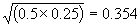## ADDENDUM The Geometric Mean

1. Sib Relationships : Strictly speaking one should not use the arithmetic mean to find the average of two quantities which are part of a power series, i.e. (1/2)1, (1/2)2, (1/2)3 etc. The geometric mean would be more appropriate. The geometric mean of two numbers is the square root of their product. Therefore, the geometric mean of 0.25 and 0.5 is :Although this is slightly below the arithmetic mean (0.375), the title of 'three-quarter sibs' is still a suitably descriptive label.

Appendix A - EXTENDED FORMULAE FOR THE coefficient of relationship : The figure of 0.75 for R for a single locus in both situations A and B is not the true value of the coefficient since we should have used the geometric mean. The geometric mean of 0.5 and 1.0 is 0.71, not 0.75. However, this does not invalidate the need for correction terms since it is still different from Σ(1/2)n in both cases.